Bilbao Crystallographic ServerSymmetry adapted modesOnline Help

## SAM - Calculation of Symmetry Adapted Modes

SAM computes the symmetry adapted modes in the Γ point and classifies them by their infrared, Raman and Hyper-Raman activity. This document explains step by step how the program can be used. There are two different options to use the program:
• OPTION A: Space group

• Input

• One has to introduce the space group number and select the Wyckoff positions occupied by the atoms in the structure.

• Output

• The program classifies the symmetry adapted modes in IR, Raman and Hyper-Raman modes. Moreover, there are shown which are the allowed second order IR and Raman modes. On the other hand, there is also available information about the point group such as character's table, irreps decomposition and selection rules.

• OPTION B: Structure

• Input

• One can introduce the structure data using a CIF file or filling the appropiate field, which should include:

• The number of the space group as given in the International Tables for Crystallography, Vol. A (ITA)
• The lattice parameters
• The number of independent atoms
• The independent positions of the atoms, including the labels, a sequential number, the Wyckoff positions (if they are unknown it is sufficient to use a dash "-" instead) and the atomic coordinates relative to the lattice parameters

• Output

• The program shows the classification of the symmetry adapted modes and the total number of IR, Raman and Hyper-Raman active modes.

• Some notes for the correct use of the program SAM:

1. Settings: In the step 1 of the input data there is a link of a table with all space group ITA numbers with the avaliable settings.

2. Millikan Notation: The irreps - irreducible representations - are written in Millikan notation. For the users of the program REPRES the output of this irreps are written in the same order of SAM are, in the special case of Γ point.

3. Modes: The symmetry adapted modes are calculated by the projection operator method and they are expressed in an orthoghonal basis.

4. Symbols:

• WP  →  Wyckoff position.
•  V     →  Vectorial representation.
•  A     →  Axial or Pseudo-vectorial representation.
• [V2]          →  Vectorial symmetrized squared representation.
• [V2] x V   →  The direct product of the vectorial symmetrized squared representation with the vectorial representation.

If you are interested in the procedure of the method please see the documentation.

## OPTION A: Space group

• ### Input data:

• Step 1: Fill in the space group of the crystal structure. One can introduce the number of the group as it is given in the ITA or you can click on choose and select from a menu.

•• Step 2: Check in the Wyckoff positions of the atoms occupied the structure.

•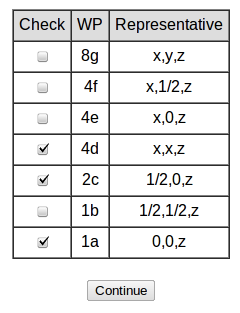• ### Output data:

• General information about the point group.

• Character tables of the point group.

•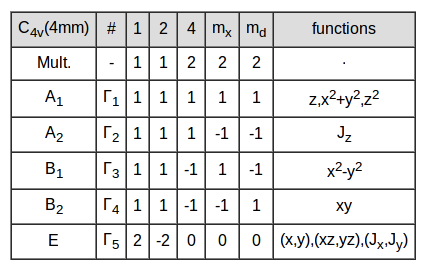The program gives access to the list of the irreducible representations in their matrix form.

• Raman and Hyper-Raman tensors.

•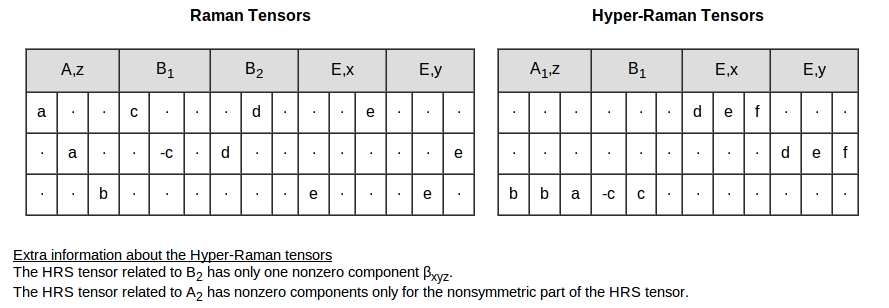There is also the option to calculate the tensors in a different orientation. The program gives access to the program TRANSFORM TENSOR.

• Information about the space group.

• Symmetry adapted modes classified by their infrared, Raman and Hyper-Raman activity.

•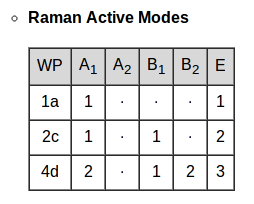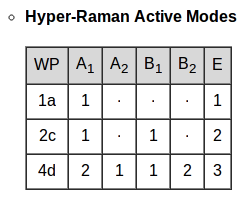• Summary table with the information about the activity of the modes.

•• Table of decompositions of the permutational representation for the different Wyckoff positions.

• The permutational representation represents the number of atoms that are invariant under the symmetry operations of the group.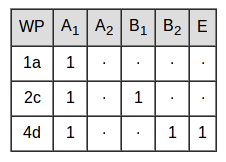• Table of decompositions of the mechanical representation for the different Wyckoff positions.

• The mechanical representation is defined as the direct product of the permutational representation with the vectorial representation.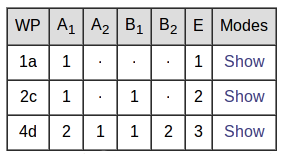Clicking in the Show option one can obtain the symmetry adapted modes for each one of the orbits.

• Decompositions of important representations such as vectorial or squared simetrized of vectorial representation.

•• Second order IR active modes:

•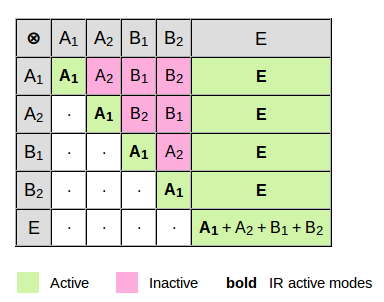For example:

• E ⊗ E = A1 + A2 + B1 + B2 is an active IR mode because the A1 mode is an IR active mode.
• A2 ⊗ B1 = B2 which is not active because this modes is not IR active.

• Second order Raman active modes:

•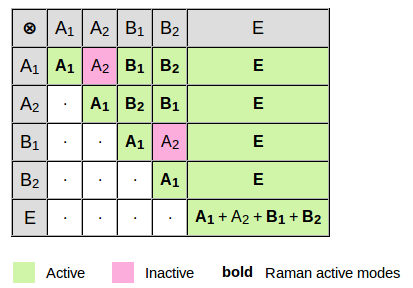For example:

• E ⊗ E = A1 + A2 + B1 + B2 is a Raman active mode because the A1 mode is a Raman active mode.
• A2 ⊗ B1 = B2 which is active because this mode is Raman active.
• B1 ⊗ B2 = A2 which is not active because this modes is not Raman active.

## OPTION B: Structure

• ### Input data:

• One hase to introduce the structure, one can do it directly using the following format:

# Space Group ITA number
167
# Lattice parameters
4.7597 4.7597 12.9935 90 90 120
# Number of independent atoms in the asymmetric unit
2
# [atom type] [number] [WP] [x] [y] [z]
Al 1 12c 0.0000 0.0000 0.3523
O 1 18e 0.3065 0.0000 0.2500

Or one can introduce the CIF file in the program.

• ### Output data:

• In the first part of the output one can find the information about the mechanical representation of the introduced structure.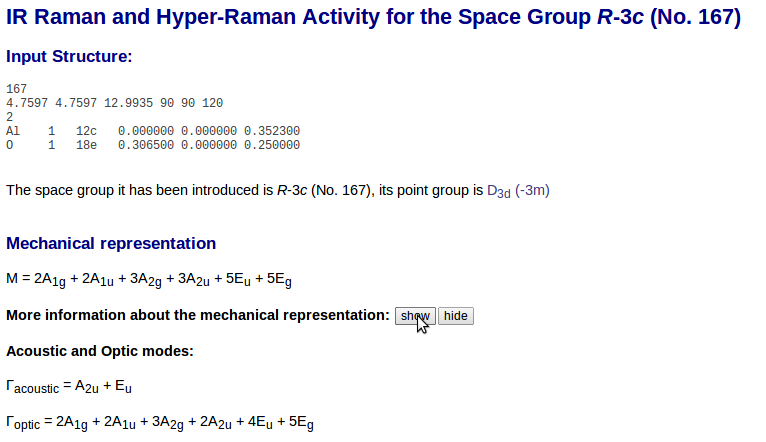After this one can find the selection rules for the structure, the IR, Raman and Hyper-Raman active modes.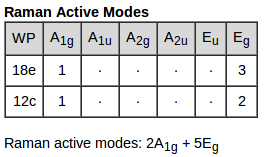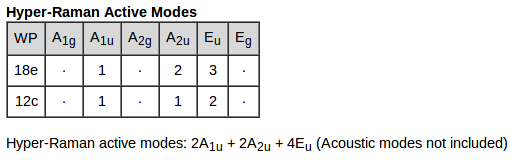The number of IR, Raman and Hyper-Raman active modes of the structure are given and one can also find the information about the acustic modes.